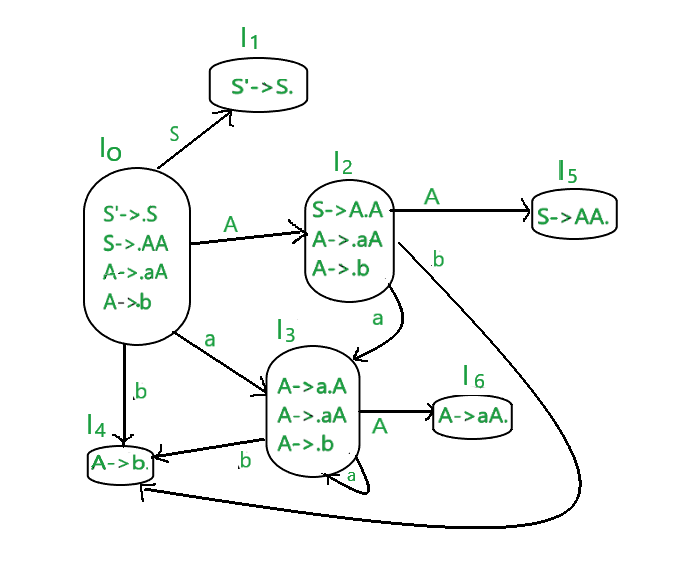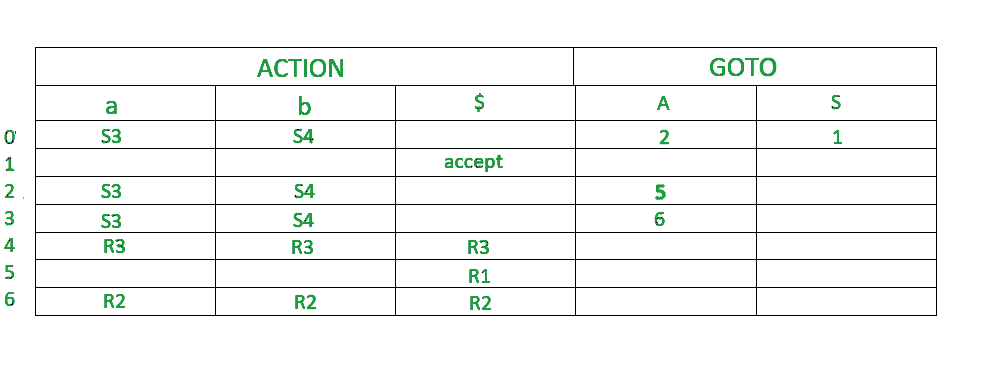Related Articles

# SLR Parser (with Examples)

• Last Updated : 03 Aug, 2021

Prerequisite : LR Parser

LR parsers :
It is an efficient bottom up syntax analysis technique that can be used to parse a large classes of  context free grammar  is called LR(0) parsing.
L stands for left to right scanning
R stands for rightmost  derivation in reverse
0 stands for no. of input symbols of lookahead

• It recognises virtually  all programming language constructs for which CFG can be written
• It is able to detect syntatic errors
• It is an efficient non-backtracking shift reducing parsing method.

Types of LR parsing methods :

1. SLR
2. CLR
3. LALR

SLR Parser :
SLR is simple LR.It is the smallest class of grammar having few number of states. SLR is very easy to construct and is similar to LR parsing. The only difference between SLR parser and LR(0) parser is that in LR(0) parsing table , theres a chance of ‘shift reduced’ conflict because we are entering ‘reduce’ corresponding to all terminal states.We can solve this problem by entering ‘reduce’ corresponding to FOLLOW of LHS of production in the terminating state.This is called SLR(1) collection of items

Steps for constructing the SLR parsing table :

1. Writing augmented grammar
2. LR(0) collection of items to be found
3. Find FOLLOW of LHS of production
4. Defining 2 functions:goto[list of terminals] and action[list of non-terminals] in the parsing table

EXAMPLE – Construct LR parsing table for the given context free grammar

S–>AA
A–>aA|b

Solution:

STEP1 – Find augmented grammar
The augmented grammar of the given grammar is:-

S’–>.S    [0th production]
S–>.AA  [1st production]
A–>.aA [2nd production]
A–>.b  [3rd production]

STEP2 – Find LR(0) collection of items
Below is the figure showing the LR(0) collection of items. We will understand everything one by one.The terminals of this grammar are {a,b}.
The non-terminals of this grammar are {S,A}

RULE –
If any non-terminal has ‘ . ‘ preceding it, we have to write all its production and add ‘ . ‘ preceding each of its production.

RULE –
from each state to the next state, the ‘ . ‘ shifts to one place to the right.

• In the figure, I0 consists of augmented grammar.
• Io goes to I1 when  ‘ . ‘ of 0th production is shifted towards the right of S(S’->S.). this state is the accept state . S is seen by the compiler.
• Io goes to I2 when  ‘ . ‘ of 1st production is shifted towards right (S->A.A) . A is seen by the compiler
• I0 goes to I3 when  ‘ . ‘ of the 2nd production is shifted towards right (A->a.A) . a is seen by the compiler.
• I0 goes to I4 when  ‘ . ‘ of the 3rd production is shifted towards right (A->b.) . b is seen by the compiler.
• I2 goes to I5 when  ‘ . ‘ of 1st production is shifted towards right (S->AA.) . A is seen by the compiler
• I2 goes to I4 when  ‘ . ‘ of 3rd production is shifted towards right (A->b.) . b is seen by the compiler.
• I2 goes to I3 when  ‘ . ‘ of the 2nd production is shifted towards right (A->a.A) . a is seen by the compiler.
• I3 goes to I4 when  ‘ . ‘ of the 3rd production is shifted towards right (A->b.) . b is seen by the compiler.
• I3 goes to I6 when  ‘ . ‘ of 2nd production is shifted towards the right (A->aA.) . A is seen by the compiler
• I3 goes to I3 when  ‘ . ‘ of the 2nd production is shifted towards right (A->a.A) . a is seen by the compiler.

STEP3 –
Find FOLLOW of LHS of production

FOLLOW(S)=\$
FOLLOW(A)=a,b,\$

STEP 4-
Defining 2 functions:goto[list of terminals] and action[list of non-terminals] in the parsing table.Below is the SLR parsing table.• \$ is by default a non terminal which takes accepting state.
• 0,1,2,3,4,5,6 denotes I0,I1,I2,I3,I4,I5,I6
• I0 gives A in I2, so 2 is added to the A column and 0 row.
• I0 gives S in I1,so 1 is added to the S column and 1 row.
• similarly  5 is written in  A column and 2 row, 6 is written in A column and 3 row.
• I0 gives a in I3 .so S3(shift 3) is added to a column and 0 row.
• I0 gives b in I4 .so S4(shift 4) is added to the b column and 0 row.
• Similarly, S3(shift 3) is added on a column and 2,3 row ,S4(shift 4) is added on b column and 2,3 rows.
• I4 is reduced state as ‘ . ‘ is at the end. I4 is the 3rd production of grammar(A–>.b).LHS of this production is A. FOLLOW(A)=a,b,\$  . write r3(reduced 3) in the columns of a,b,\$ and 4th row
• I5 is reduced state as ‘ . ‘ is at the end. I5 is the 1st production of grammar(S–>.AA). LHS of this production is S.
FOLLOW(S)=\$  . write r1(reduced 1) in the column of \$ and 5th row
• I6 is reduced state as ‘ . ‘ is at the end. I6 is the 2nd production of grammar( A–>.aA). The LHS of this production is A.
FOLLOW(A)=a,b,\$  . write r2(reduced 2) in the columns of a,b,\$ and 6th row

Attention reader! Don’t stop learning now.  Practice GATE exam well before the actual exam with the subject-wise and overall quizzes available in GATE Test Series Course.

Learn all GATE CS concepts with Free Live Classes on our youtube channel.

My Personal Notes arrow_drop_up